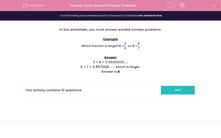# Solve Worded Number Problems

In this worksheet, students solve worded number problems with or without the aid of a calculator.This content is premium and exclusive to EdPlace subscribers.Key stage:  KS 2

Curriculum topic:   Maths and Numerical Reasoning

Curriculum subtopic:   Mixed Problems

Difficulty level:#### Worksheet Overview

In this worksheet, you must answer worded number problems.

Example

Which fraction is larger? A =
 3 9
or B =
 6 7

3 ÷ 9 = 0.33333333.....

6 ÷ 7 = 0.8571428......, which is larger.